## RS Aggarwal Class 8 Solutions Chapter 2 Exponents Ex 2B

These Solutions are part of RS Aggarwal Solutions Class 8. Here we have given RS Aggarwal Solutions Class 8 Chapter 2 Exponents Ex 2B.

Other Exercises

Question 1.
Solution:
(i) 57.36 = 5.736 x 101
(ii) 3500000 = 3.5 x 106
(iii) 273000 = 2.73 x 105
(iv) 168000000 = 1.68 x 108
(v) 4630000000000 = 4.63 x 1012
(vi) 345 x 105 = 3.45 x 102 x 105 = 3.45 x 107

Question 2.
Solution:
(i) 3.74 x 105
= $$\\ \frac { 374 }{ 100 }$$ x 105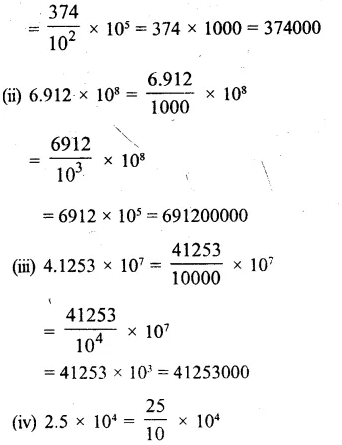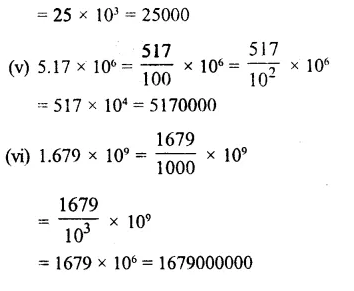Question 3.
Solution:
(i) Height of Mount Everest = 8848 m
= 8.848 x 1000
= 8.848 x 103
(ii) Speed of light = 300000000 m/sec.
= 3.00000000 x 100000000
= (3 x 108) m/sec.
(iii) Distance between the earth and the sun = 149600000000 m
= (1.49600000000 x 100000000000)m = (1.496 x 1011) m

Question 4.
Solution:
Mass of earth = (15.97 x 1024) kg
and mass of moon = (7.35 x 1022) kg
= Total mass of earth and moon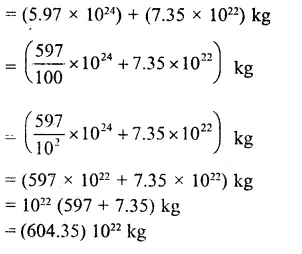Question 5.
Solution:
(i) 0.0006
= $$\\ \frac { 6 }{ 10000 }$$ = $$\frac { 6 }{ { 10 }^{ 4 } }$$ = 6.10-4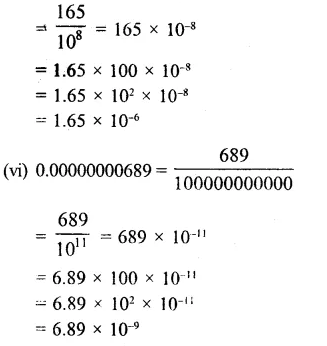Question 6.
Solution:Question 7.
Solution:
(i) 2.06 x 10-5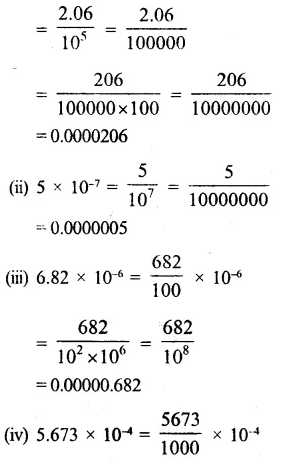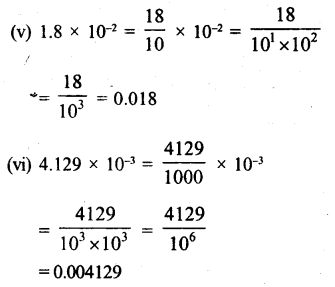Hope given RS Aggarwal Solutions Class 8 Chapter 2 Exponents Ex 2B are helpful to complete your math homework.

If you have any doubts, please comment below. Learn Insta try to provide online math tutoring for you.